The Banach-Tarski Paradox is a theorem in geometry which states that:

“It is possible to decompose a ball into five pieces which can be reassembled by rigid motions to form two balls of the same size as the original.”

It was first stated in 1924, and is called a paradox as it contradicts basic geometric intuition.

An alternate version of this theorem tells us that:

“It is possible to take a solid ball the size of a pea, and by cutting it into a finite number of pieces, reassemble it to form a solid ball the size of the sun.”

Below is an awesome video explaining how this paradox works:

M x

## Sublime Symmetry

The De Morgan Foundation organised a one day synopsium called ‘Sublime Symmetry’ on the 13th January, which explored the mathematics behind William De Morgan’s ceramic designs.

William De Morgan was a ceramic designer in the late Victorian period.

“His conjuring of fantastical beasts to wrap themselves around the contours of ceramic hollowware and his manipulation of fanciful flora and fauna to meander across tile panels fascinated his contemporaries and still captivates today.

The ‘Sublime Symmetry’ exhibition highlights the influence of geometry in William De Morgan’s work, and particularly the use of symmetry to create his designs. This application of geometry naturally produces beautiful and visually striking images. Below are some images of his work:I find it fascinating how mathematics is so naturally interlaced in art and beauty, and so I really wanted to share this with you. Hopefully you found it interesting as well! The ‘Sublime Symmetry’ exhibition will be at the New Walk Gallery in Leicester until the 4th March, after which it will be displayed at the William Morris Gallery in Walthamstow on the 12th March until the 3rd September. [Source]

M x

## Maths and Biology: Viruses

Viruses are effective carriers of information. This information is called RNA (sort of like single stranded DNA), which is held in a vessel called the capsid. As it is favourable for the virus to be as efficient as possible, the capsid is made up of a repeating structure of proteins, which is coded by the RNA which it holds. Due to this repeating structure, the capsid has rotational symmetry.

These constraints on the organisation of the capsid proteins mean that they can only have helical or spherical structures. Thus, there are only a finite number of symmetries that they can have in 3-dimensions, which are embodied by the Platonic Solids.

Most viruses exhibit icosahedral symmetry, meaning they have the same properties as the icosahedron:

• formed of 20 triangles
• 60 rotational symmetries

Another example is the common cold and HPV (Human papillomavirus), which are both caused by viruses of different sizes, but have the same symmetry: 6-fold rotational symmetry.

However, a more interesting group of viruses are those with 5-fold symmetry, as the structure of these is much harder to determine.

### Structure via Symmetry

Symmetry is the key to finding the structure of a viruses, and as they are too small to see in microscopes, their structure can be determined using a technique called Cryo-electon microscopy, which is a bit like unfolding the shape and laying it out flat.

For structures with a 5-fold symmetry axis, this technique is almost impossible to use. An analogy of this is “trying to tile a bathroom floor: it is possible with hexagons but not with pentagons.” This mathematical phenomenon means that it is therefore necessary to know the overall symmetry of structures with 5-fold symmetries before their structure can be determined using cryo-EM.

But what is the reason for all this symmetry?

“Symmetry goes hand in hand with stability. Symmetric configurations are usually more stable, a feature that is important for viruses when transporting their genomic material between hosts.”

Source

A bit of a different topic today. Let me know if you like it! M x

## New Books in Maths: October 2016 (II)

The Circle: A Mathematical Exploration Beyond the Line – Alfred S. Posamentier and Robert Geretschlager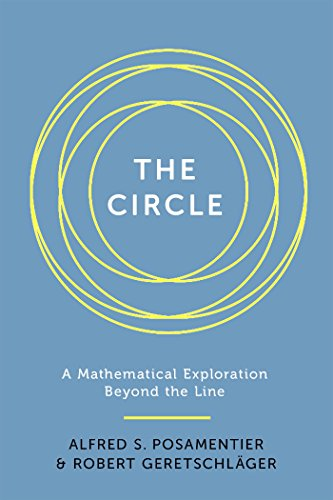The circle has played a pivotal role in our society, appearing in our art, literature, and culture and has helped develop our industrial civilisation. So, it is no surprise that the circle has fascinated mathematicians since the ancient times.

This book aims to underline the many intriguing properties of the circle to beginners by describing how the circle “manifests itself in the field of geometry”, leading to important relationships and theorems. It also highlights how the circle continues to charm mathematicians today, as they continue to discover new and surprising properties about this most fundamental of shapes.

Elements of Mathematics: From Euclid to Gödel – John Stillwell

Elements of Mathematics takes readers on a journey of elementary mathematics, which as Stillwell shows is not as simple as one may believe. As he examines elementary mathematics from a 21st century standpoint, the beauty and scope of the discipline, as well as its limits, are revealed.

From Gaussian integers to propositional logic, Stillwell delves into arithmetic, computation, algebra, geometry, calculus, combinatorics, probability, and logic.” He describes how the research in these areas helped build the foundations of, and hence develop, modern mathematics into what it is today.

This is not Stillwell’s first book; apart from being a professor of mathematics at the University of San Francisco he has also written many books including ‘Mathematics and Its History’ and ‘Roads to Infinity’.

For those of you interested in the history of mathematics, this is a must read!

“An accessible read. . . . Stillwell breaks down the basics, providing both historical and practical perspectives from arithmetic to infinity.” – Gemma Tarlach, Discover

Read part 1 here! M x

## Unsolved Problems: Part II

This is a continuation of my post from last Thursday on simple unsolved problems in mathematics.

### Inscribed Square Problem

The inscribed square problem is an unsolved problem in geometry, proposed by German mathematician Otto Toeplitz in 1911. It asks whether it is possible to draw a square inside a closed loop so that all four corners of the square are contained in this closed curve.

Some early positive results were obtained by Arnold Emch and Lev Schnirelmann, but the general case still remains open. An example of a positive result is shown below:This has already been solved for other shapes, such as triangle and rectangles, but the case with squares is proving trickier to solve.

### Happy Ending Problem

The Happy Ending problem, so named by Paul Erdős because it led to the marriage of Esther Klein and George Szekeres, is the following statement:

“Theorem: any set of five points in the plane in general position has a subset of four points that form the vertices of a convex quadrilateral.”

In other words, if you make five dots on a piece of paper, you should always be able to connect four of them to create a convex quadrilateral (i.e. a shape with four sides where all the corners are less than 180 degrees) regardless of the position of these dots.

Although the result for a four sided shape is known (and in fact it is known for a pentagon – 9 dots are needed – and a hexagon – 17 dots are needed), it is still unknown how many dots is needed to create a shape with a greater number of sides.

Mathematicians speculate that the equation which determines the number of dots M can be related to the number of sides in the shape N by the following equation:

M=1+2N-2

However, it has only yet been proved that this will give a number AT LEAST as big as the answer.

Hope you enjoyed this mini-series! Let me know if you want me to continue it. M x

## Borromean Rings

Borromean rings are said to have originated from Afghan Buddhist art in the 2nd century, but realistically they could date back to earlier civilisations.In 15th century Italy, the Borromeo family’s crest included this pattern of three intertwining rings; the rings were “depicted in such a way so that if one of the rings were cut, all three would separate, implying greater strength through unity“. This feature is called a Brunnian link, i.e. removing any ring results in two unlinked rings.

These rings have been discovered to occur at a molecular level in DNA and in other nanotechnology research, where they are linked in 3-dimensions rather than on a flat plane.

Although the typical picture of Borromean rings leads us to believe that this link can be formed from geometrically ideal circles, or in other words constructed using three circular rings of either equal or differing radii, in fact it turns out that this cannot be. However, they can be made from three congruent elliptical rings, whose eccentricity may be taken to be arbitrarily small, i.e. “no matter how close to being circular their shape may be, as long as they are not perfectly circular“.

Update: I think I’m going to change my schedule to Monday and Thursday! I think 2 posts a week is more manageable due to my new university schedule…

M x

## Isoperimetric inequality

The isoperimetric inequality dates back to the olden days, where there was a problem that asked: “Among all closed curves in the plane of fixed perimeter, which curve (if any) maximises the area of its enclosed region?” This is equivalent to asking: “Among all closed curves in the plane enclosing a fixed area, which curve (if any) minimises the perimeter?” The solution to this problem is expressed in the form of an inequality: the isoperimetric inequality.

The isoperimetric inequality is a geometric inequality that involves the square of the circumference of a closed curve in a plane and the area that it encloses in that plane. This inequality states thatwhere L is the length of a closed curve and A is the area of the planar region that it encloses.

Many proofs have been published for this inequality. For example, in 1938 E. Schmidt produced an elegant proof based on “the comparison of a smooth simple closed curve with an appropriate circle”.

An extension of this inequality is the isoperimetric quotient, Q, of a closed curve which is defined in the following way:Hence, it is the ratio of its area and that of a circle with the same perimeter.

The inequality highlights how  Q ≤ 1 and for a circle Q = 1.

M x

## NEWS: Hitchin wins Shaw Prize

This years Shaw Prize in mathematics was awarded to Professor Nigel Hitchin, from the University of Oxford. This award was honoured to him due to “his far reaching contributions to geometry, representation theory and theoretical physics. The fundamental and elegant concepts and techniques that he has introduced have had wide impact and are of lasting importance.”Hitchin’s innovative work has had a profound impact on a variety of areas in Mathematics, including algebraic geometry, differential geometry, complex analysis, topology, integrable systems, mathematical and theoretical physics.

One of his most notable achievements was deriving the self-duality equations, which are a “special class of solutions of the self-dual Yang-Mills equations“. He discovered that when described on a compact Riemann surface – a surface that can be covered by a finite collection of planes –  a “surprisingly rich space of solutions” is produced.

A typical feature of Hitchin’s work is as follows: he proves that objects in theoretical physics define new concepts in algebraic or differential geometry, and then uses rigorous mathematics to produce powerful and elegant results.

Marta Mazzocco describes how

… Hitchin defies the stereotype that mathematicians need to be in their early thirties to produce great results. When already in his sixties, Hitchin introduced the notion of co-Higgs bundle …“.

An apt winner of the Shaw Prize, I hope to hear of his work for many years to come.

M x

## Maths of Origami

Origami originated in China, although it is now associated to Japan, around 200AD and was then known as Zhezhi. It was brought to Japan in the 6th century by Chinese Buddhist monks, where it obtained the name origami (”ori’ meaning fold and ‘gami’ meaning paper). Paper folding has become of great interest to mathematicians and in today’s post I will discuss the mathematics behind it.

### Flat Folding

The construction of origami models is often shown by the patterns left by the creases. The main question that mathematicians are interested in is looking at these crease patterns and answering whether they can be folded to a flat model, and if so, how to fold them. There are three rules for making flat-foldable origami crease patterns:

1. Maekawa’s Theorem: at any vertex the number of valley (crease goes inwards) and mountain (crease goes outwards) folds always differs by two;
2. Kawasaki’s Theorem: if you add up the angle measurements of every other angle around a point, the sum will be 180 degrees;
3. A sheet can never penetrate a fold.

### Huzita-Hatori Axioms

Just as Euclid devised axioms for planar geometry, Humiaki Huzita and Koshiro Hatori created a complete set of axioms to describe the geometry of origami.

• Given two points p1 and p2, there is a unique fold that passes through both of them;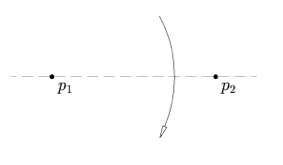• Given two points p1 and p2, there is a unique fold that places p1 into p2;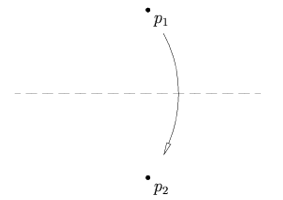• Given two lines l1 and l2, there is a fold that places l1 onto l2;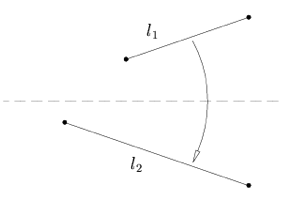• Given a point p1 and a line l1, there is a unique fold perpendicular to l1 that passes through point p1;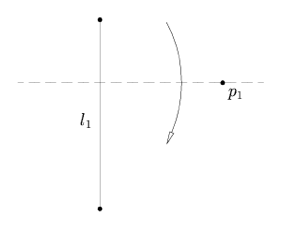• Given two points p1 and p2 and a line l1, there is a fold that places p1 onto l1 and passes through p2;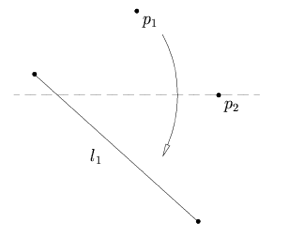• Given two points p1 and p2 and two lines l1 and l2, there is a fold that places p1 onto l1 and p2 onto l2;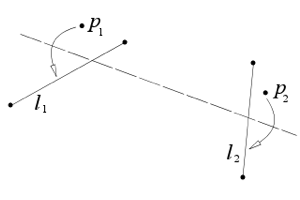• Given one point p and two lines l1 and l2, there is a fold that places p onto l1 and is perpendicular to l2.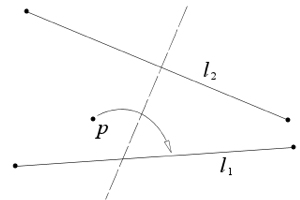[Taken from plus.maths.org]

### Constructions

Origami has also been applied to solve geometric problems, which cannot be solved using a compass and unmarked ruler. I will highlight a few examples.

Doubling the Cube

This problem concerns a cube with a side length s1 and of volume V. The challenge is to find a cube with side length s2, such that its volume is now 2V. This construction is credited to Peter Messer and goes as follows:

Trisecting an angle

This construction is due to Hisashi Abe.

Any suggestions for future posts? M x

## Euclid’s Axioms

Euclid’s book Elements is the earliest known systematic discussion on geometry. In it he laid the foundations and rules to what is now known as Euclidean geometry. In Elements, Euclid assumed a small set of axioms, from which theorems could be deduced. So what are these axioms?

#### Given any two points, you can draw a straight line between them, forming a line segment.#### Given a point and a line segment starting at the point, you can draw a circle centred on the given point with the given line segment as its radius.#### All right angles are equal to each other.